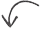# Converting dBm to Watts

In the field of electrical engineering, power is most often specified in dBm–not Watts.  This brief post talks about how to convert between dBm and Watts and what the dBm unit means.

A complete explanation of the decibel and log / linear conversions for many units (including dBm) can be found in The Decibel article.

dBm and Watts ConversionNotice that the equations are for mW (milliwatts), not Watts.  That’s because the dBm is a unit based on the mW.  Converting to mW to W is simply done by multiplying by 1000, since there are 1000 mW in a Watt.

## Why Use dBm?

Electrical engineers use dBm most often for power specifications because it’s much easier to work with large numbers using the log scale instead of the linear scale.  The dBm is a unit based on the log scale, and can be simply used with other design components with gains and losses specified in dB.

Check out The Decibel article for more complete explanation of why we use dB instead of linear units.  The Decibel article also explains many other common units based on the dB, and how to convert between their log and linear equivalents.

If you found this post helpful, you can let us know by tweeting us @bitweenie or me at @shilbertbw or leaving a comment right here!

## Related content:Did you enjoy this article?Share
the
Love